# Trigonometric Ratios of Arbitrary Angles

Trigonometric Ratios of Arbitrary Angles
Go back to  'Trigonometric Ratios'

## Introduction:

In your first exposure to trigonometry, you have dealt with trigonometric ratios of angles between 00 and 900. However, in general, we can talk about trigonometric ratios of angles of arbitrary magnitudes (any magnitude).
Let us see how.

## Trigonometric ratios of arbitrary angles:

Let's try to understand it by dividing the coordinate axes system (anticlockwise) manner into four quadrants - 1st, 2nd, 3rd, and 4th quadrant.

### Angles in $${\rm I}$$ Quadrant:

The following figure shows a unit circle centered at the origin of a coordinate axes system.

A variable point $$P$$ moves on the circumference of this circle. In the figure below, $$P$$ is in the first quadrant, and $$OP$$ makes an acute angle of $$\theta$$ radians with the positive $$x$$-direction. $$PQ$$ is the perpendicular dropped from $$P$$ onto the horizontal axis: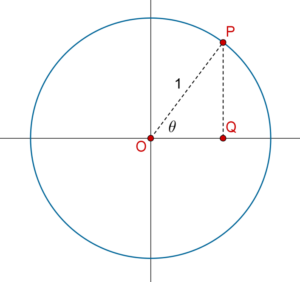It is straight forward to define the trigonometric ratios of theta in this configuration.
For example,

\begin{align}&\sin \theta = \frac{{PQ}}{{OP}} = PQ\\&\tan \theta = \frac{{PQ}}{{OQ}}\end{align}

Note the following special cases:

1. $$\theta = 0$$. If $$P$$ is on the positive $$x$$-axis, then $$\theta = 0$$. In this configuration, the perpendicular $$PQ=0$$ while the base $$OQ$$ and hypotenuse $$OP$$ equal to 1 (basically, $$P$$ and $$Q$$ coincide).
Thus,

$\sin 0 = 0,\,\,\,\cos 0 = 1,\,\,\,\tan 0 = 0$

2. $$\theta = \frac{\pi }{2}\,\,{\rm{or}}\,\,{90^0}$$. If $$P$$ is on the positive $$y$$-axis, then $$\theta = \frac{\pi }{2}$$ radians, and the perpendicular $$PQ$$ = hypotenuse $$OP$$ = 1, while the base $$OQ$$ = 0: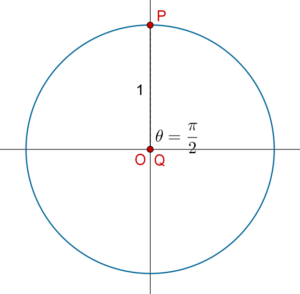Thus,

$\sin \frac{\pi }{2} = 1,\,\,\,\cos \frac{\pi }{2} = 0,\,\,\,\tan \frac{\pi }{2}:{\rm{N.D.}}$

### Angles in $${\rm I}{\rm I}$$ Quadrant:

Now, suppose that $$P$$ moves into the second quadrant, so that $$\theta$$ is now an obtuse angle (between $$90^\circ$$ and $$180^\circ$$):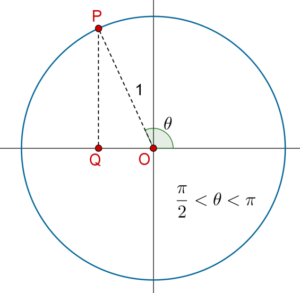Can we define the trigonometric ratios for $$\theta$$ now? Yes! We still consider $$PQ$$ as our perpendicular, $$OQ$$ as our base and $$OP$$ as the hypotenuse, so for example:

\begin{align}&\cos \theta = \frac{{OQ}}{{OP}} = OQ\\&\cot \theta = \frac{{OQ}}{{PQ}}\end{align}

Note: We must take $$OQ$$ to be negative, since it is in the negative $$x$$-direction. This means that $$\cos \theta$$, $$\sec \theta$$, $$\tan \theta$$ and $$\cot \theta$$ will be negative. However, $$\sin \theta$$ and $$\text{cosec}\, \theta$$ will still be positive.

When $$P$$ is on the negative $$x$$-axis, $$\theta$$ is exactly $$\pi$$ radians: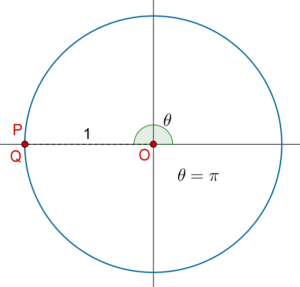Note that $$PQ$$ has reduced to 0, while $$OQ$$ = $$OP$$ = 1, so that,

$\sin \pi = 0,\,\,\,\cos \pi = - 1,\,\,\,\tan \pi = 0$

### Angles in $${\rm I}{\rm I}{\rm I}$$ Quadrant:

When $$P$$ is in the third quadrant, $$\theta$$ is between $$180^\circ$$ and $$270^\circ$$. Both the perpendicular and base will be taken as negative in this configuration: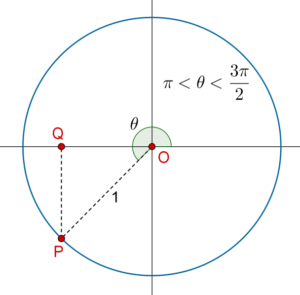The trigonometric ratios will still be defined using $$PQ$$ as the perpendicular, $$OQ$$ as the base and $$OP$$ as the hypotenuse. For example:

\begin{align}&\cot \theta = \frac{{OQ}}{{PQ}}\\&\cos \theta = \frac{{OQ}}{{OP}} = OQ\end{align}

Note: $$\sin \theta$$, $$\text{cosec}\, \theta$$, $$\cos \theta$$ and $$\sec \theta$$ will be negative, while $$\tan \theta$$ and $$\cot \theta$$ will be positive.

When $$P$$ is on the negative $$y$$-axis, theta is exactly $$270^\circ$$ or $$\frac{{3\pi }}{2}$$ radians. In this configuration, the base $$OQ$$ has become 0, but the perpendicular $$PQ$$ = the hypotenuse $$OP$$ = 1: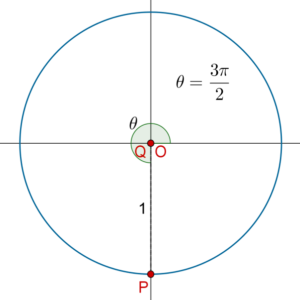Thus,

$\sin \frac{{3\pi }}{2} = - 1,\,\,\,\cos \frac{{3\pi }}{2} = 0,\,\,\,\tan \frac{{3\pi }}{2}:{\rm{nd}}$

### Angles in $${\text{IV}}$$ Quadrant:

When $$P$$ is the fourth quadrant, $$\theta$$ is between $$270^\circ$$ and $$360^\circ$$. The perpendicular is negative but the base is positive in this configuration: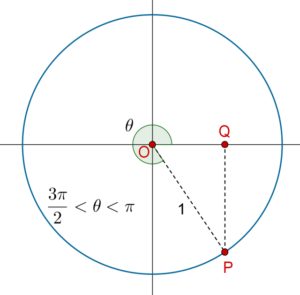Once again, we will use $$PQ$$ as the perpendicular, $$OQ$$ as the base and $$OP$$ as the hypotenuse to determine the trigonometric ratios for $$\theta$$. For example:

\begin{align}&\sin \theta = \frac{{PQ}}{{OP}} = PQ\\&\cos \theta = \frac{{OQ}}{{OP}} = OQ\end{align}

Note: In this configuration, $$\sin \theta$$, $$\text{cosec}\, \theta$$, $$\tan \theta$$ and $$\cot \theta$$ will be negative, while $$\cos \theta$$ and $$\sec \theta$$ will be positive.

### Angles greater than $$360^\circ$$:

When $$P$$ comes back on the positive $$x$$-axis, we have $$\theta \,\, = {360^0}$$, but this is the same configuration as $$\theta = {\rm{ }}{0^0}$$, which means that the trigonometric ratios of $$360^\circ$$ will be the same as the trigonometric ratios of $$0^\circ$$.

What if $$\theta$$ increases further now? For example, suppose that you are asked to calculate the trigonometric ratios of $$390^\circ$$. What will you do?

Note that

${390^0} = {360^0} + {30^0}$

This means that $$\theta = {\rm{ }}{390^0}$$ represents exactly the same geometrical configuration as $$\theta = {\rm{ }}{30^0}$$: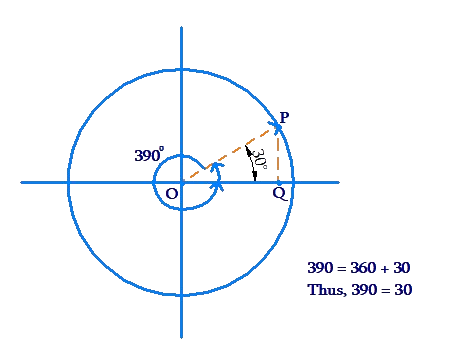Thus, the trigonometric ratios of $$390^\circ$$ will be exactly the same as the trigonometric ratios of $$30^\circ$$. For example,

\begin{align}&\tan {390^0} = \tan {30^0} = \frac{1}{{\sqrt 3 }}\\&\sin {390^0} = \sin {30^0} = \frac{1}{2}\end{align}

What about the trigonometric ratios of $$1100^\circ$$? Note that

\begin{align}{1100^0} &={1080^0} + {20^0}\\\qquad&= \left( {3 \times {{360}^0}} \right) + {20^0}\end{align}

Thus, $$1100^\circ$$ represents the same geometrical configuration as $$20^\circ$$ (since $$1100^\circ$$ is three complete revolutions plus $$20^\circ$$):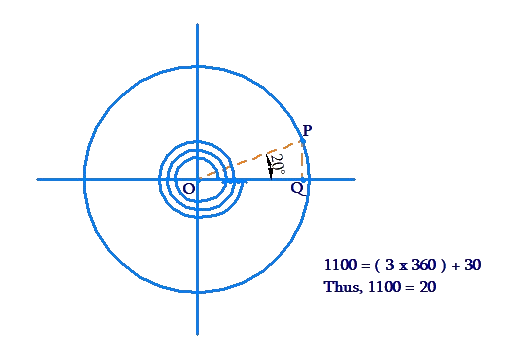This means that the trigonometric ratios of $$1100^\circ$$ will be the same as the trigonometric ratios of $$20^\circ$$.

This way, we can calculate the trigonometric ratios of angles of arbitrary positive magnitudes. Given any positive angle $$\theta$$, we determine the geometrical configuration which $$\theta$$ represents – we find the corresponding angle $$\varphi$$ in the range $$\left[ {{0^0},{{360}^0}} \right]$$. The trigonometric ratios of $$\theta$$ will be exactly the same as the trigonometric ratios of $$\varphi$$.

But what about negative angles? For example, what are the trigonometric ratios of $$- {30^0}$$, or $$- \frac{\pi }{6}$$ rad? Does this question make any sense? Let's see:

### Trigonometric ratios of negative angles:

Yes. Once again, we think from the perspective of geometrical configurations. What geometrical configuration does an angle of $$- {30^0}$$ represent? Since positive angles were measured in the anti-clockwise direction, we can associate negative angles with the clockwise direction. Thus, an angle of $$- {30^0}$$ can be thought of as follows: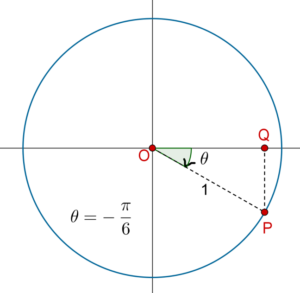But this is the same as $$330^\circ$$. Thus, the trigonometric ratios of $$-30^\circ$$ is the same as the trigonometric ratios of $$330^\circ$$. This fits because the difference between the two angles is $$360^\circ$$:

${330^0} - {\left( { - 30} \right)^0} = {360^0}$

Thus, the two angles are equivalent, in the sense that they represent the same direction.

The following figure shows an angle of $$- {120^0}$$ or $$- \frac{{2\pi }}{3}$$ radians: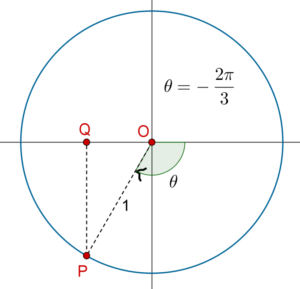This angle is the same as a positive angle of $$240^\circ$$ or $$\frac{{4\pi }}{3}$$ radians. Hence the trigonometric ratios of $$- {120^0}$$ will be the same as the trigonometric ratios of $$240^\circ$$. Also, note that

${240^0} - {\left( { - 120} \right)^0} = {360^0}$

What configuration does an angle of $$- {1000^0}$$ represent? We note that

$- {1000^0} = - {720^0} - {280^0}$

This corresponds to the following configuration: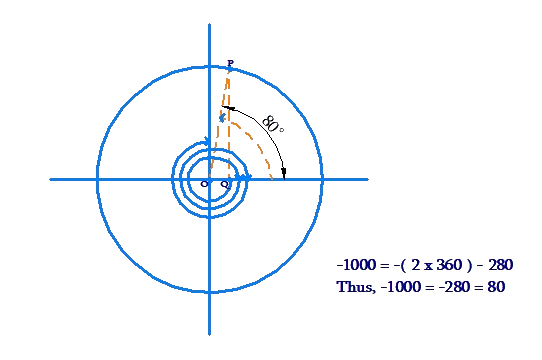We see that $$- {1000^0}$$ actually represents the same direction as $$80^\circ$$. This is also evident from the following equation:

\begin{align} - {1000^0} &= - {1080^0} + {80^0}\\\qquad\qquad&= - \left( {3 \times {{360}^0}} \right) + {80^0}\\ \Rightarrow - {1000^0} &\equiv {80^0}\end{align}

Thus, the geometrical configuration corresponding to $$- {1000^0}$$ can be obtained by taking 3 clockwise revolutions of $$360^\circ$$, and then an anti-clockwise sweep of $$80^\circ$$: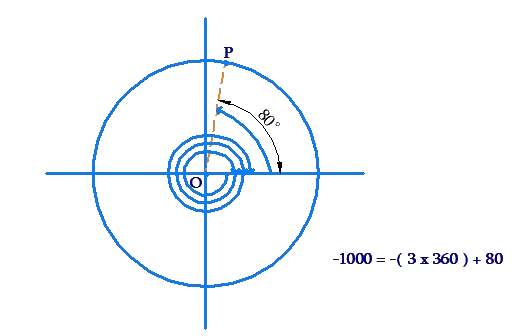Note: This way, we can define trigonometric ratios of negative angles of arbitrary angles as well. Thus, we conclude that trigonometric ratios can be defined for angles with any real value. Given an arbitrary angle, say $$x$$ radians, we need to find the geometrical configuration which that angle represents. Then, we can find the perpendicular, base and hypotenuse corresponding to $$x$$, and calculate all the trigonometric ratios.

## Solved Example:

Example 1: Find the values of

a) $$\sin {780^0}$$

b) $$\cos \left( { - {{660}^0}} \right)$$

c) $$\tan \left( {\frac{{41\pi }}{4}} \right)$$

d) $$\cot \left( { - \frac{{19\pi }}{3}} \right)$$

Solution: (a) We have:

\begin{align}{780^0} &= {720^0} + {60^0}\,\,\, \\\Rightarrow \,\,\,{780^0} &\equiv {60^0}\\ \Rightarrow \,\,\,\sin \left( {{{780}^0}} \right) &= \sin \left( {{{60}^0}} \right) \\&= \boxed{\frac{{\sqrt 3 }}{2}}\end{align}

(b) We note that:

\begin{align}- {660^0} &= - {720^0} + {60^0}\\ \Rightarrow - {660^0} &\equiv {60^0}\\ \Rightarrow \cos \left( { - {{660}^0}} \right) &= \cos \left( {{{60}^0}} \right)\\ &= \boxed{\frac{1}{2}}\end{align}

(c) We have:

\begin{align} \frac{{41\pi }}{4} &= 10\pi + \frac{\pi }{4}\,\,\, \hfill \\ \Rightarrow \frac{{41\pi }}{4} &\equiv \frac{\pi }{4} \hfill \\ \Rightarrow \tan \left( {\frac{{41\pi }}{4}} \right) &= \tan \frac{\pi }{4} \hfill \\ &= \boxed{1} \hfill \\ \end{align}

(d) We have:

\begin{align} - \frac{{19\pi }}{3} &= - 6\pi - \frac{\pi }{3}\,\,\, \hfill \\ \Rightarrow - \frac{{19\pi }}{3} &\equiv - \frac{\pi }{3} \hfill \\ \Rightarrow \cot \left( { - \frac{{19\pi }}{3}} \right) &= \cot \left( { - \frac{\pi }{3}} \right) \hfill \\ &=\boxed { - \sqrt 3} \hfill \\ \end{align}

Can you see how the value in the last step was obtained?Challenge: Find the values of:

e) $$\sec\left( {\frac{{17\pi }}{6}} \right)$$

f) $$\text{cosec}\,\left( { - \frac{{29\pi }}{3}} \right)$$

Tip: Use a similar approach as in example-1.

Trigonometry
Trigonometry
Grade 9 | Questions Set 1
Trigonometry
Grade 10 | Questions Set 1
Trigonometry